# Randomness Assumption (IID assumption)

The randomness assumption (also known as the IID assumption) is that the observations in a sequence are generated independently from the same probability distribution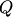on the space of possible observations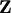(often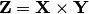). A weaker (for a wide class of, according to de Finetti's theorem) assumption is that of exchangeability.

The randomness assumption is used in stochastic prediction and conformal prediction. It is a standard assumption in machine learning. In applications, algorithms developed under this assumption (such as SVM) are often applied when the assumption is violated. However, if the observations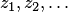,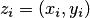, are coming from a stationary measure on, the IID assumption can be often made "almost satisfied" by extending the objects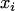. For example, in the case of time series we may add the pre-history of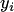to(and this will work very well if the time series is Markov).